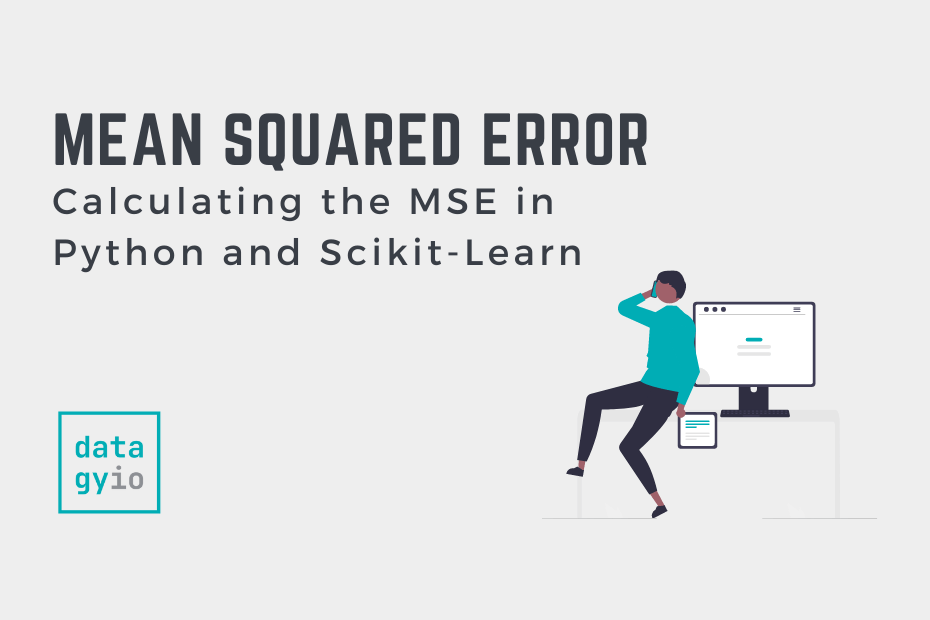# Machine Learning## How to Calculate Mean Squared Error in Python

The mean squared error is a common way to measure the prediction accuracy of a model. In this tutorial, you’ll learn how to calculate the mean squared error in Python. You’ll start off by learning what the mean squared error… Read More »How to Calculate Mean Squared Error in Python## Splitting Your Dataset with Scitkit-Learn train_test_split

In this tutorial, you’ll learn how to split your Python dataset using Scikit-Learn’s train_test_split function. You’ll gain a strong understanding of the importance of splitting your data for machine learning to avoid underfitting or overfitting your models. You’ll also learn… Read More »Splitting Your Dataset with Scitkit-Learn train_test_split## Linear Regression in Scikit-Learn (sklearn): An Introduction

In this tutorial, you’ll learn how to learn the fundamentals of linear regression in Scikit-Learn. Throughout this tutorial, you’ll use an insurance dataset to predict the insurance charges that a client will accumulate, based on a number of different factors. You’ll… Read More »Linear Regression in Scikit-Learn (sklearn): An Introduction## Introduction to Random Forests in Scikit-Learn (sklearn)

In this tutorial, you’ll learn what random forests in Scikit-Learn are and how they can be used to classify data. Decision trees can be incredibly helpful and intuitive ways to classify data. However, they can also be prone to overfitting,… Read More »Introduction to Random Forests in Scikit-Learn (sklearn)## Calculate the Pearson Correlation Coefficient in Python

In this tutorial, you’ll learn how to calculate the Pearson Correlation Coefficient in Python. The tutorial will cover a brief recap of what the Pearson correlation coefficient is, how to calculate it with SciPy and how to calculate it for… Read More »Calculate the Pearson Correlation Coefficient in Python## How to Calculate a Z-Score in Python (4 Ways)

In this tutorial, you’ll learn how to use Python to calculate a z-score for an array of numbers. You’ll learn a brief overview of what the z-score represents in statistics and how it’s relevant to machine learning. You’ll then learn… Read More »How to Calculate a Z-Score in Python (4 Ways)## Normalize a Pandas Column or Dataframe (w/ Pandas or sklearn)

Learn how to normalize and standardize a Pandas Dataframe with sklearn, including max absolute scaling, min-max scaling and z-scoare scaling.## Pandas get_dummies (One-Hot Encoding) Explained

The pandas get dummies function allows you to easily one-hot encode your data sets for use in machine learning algorithms.